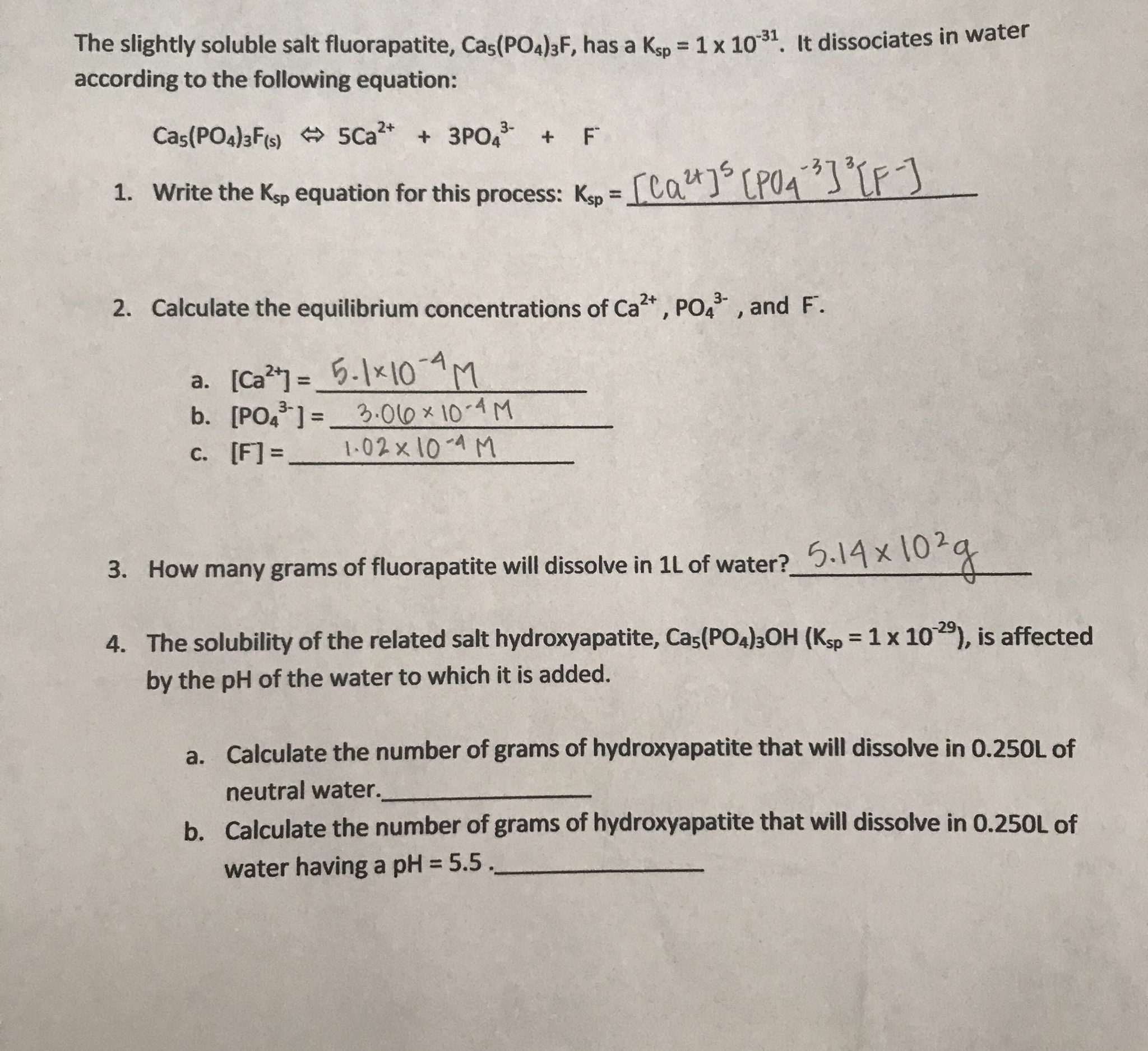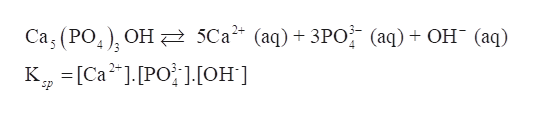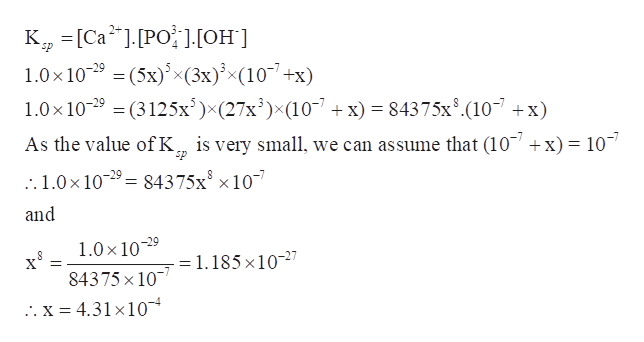# The slightly soluble salt fluorapatite, Cas(PO4)3F, has a Kso 1x 1031. It dissociates in wateraccording to the following equation:Cas(PO4)3Fi) 5Ca 3PO4 FFCP041. Write the Kp equation for this process: K =Ca3-Calculate the equilibrium concentrations of Ca, PO4, and F.2.a. [Ca]5-1x10 4M[PO 2.00x 10-4M1-02x 10-4 Mc. [F]=3. How many grams of fluorapatite will dissolve in 1L of water? .14X4. The solubility of the related salt hydroxyapatite, Cas(PO4)3OH (Ksp 1x 10), is affectedby the pH of the water to which it is added.Calculate the number of grams of hydroxyapatite that will dissolve in 0.250L ofa.neutral water.Calculate the number of grams of hydroxyapatite that will dissolve in 0.250L ofb.water having a pH 5.5

Question
22 views

I need help with #4. I think all the previous answers are correct, I'm just not sure how to do #4, both a. and b.help_outlineImage TranscriptioncloseThe slightly soluble salt fluorapatite, Cas(PO4)3F, has a Kso 1x 1031. It dissociates in water according to the following equation: Cas(PO4)3Fi) 5Ca 3PO4 F F CP04 1. Write the Kp equation for this process: K =Ca 3- Calculate the equilibrium concentrations of Ca, PO4 , and F. 2. a. [Ca]5-1x10 4M [PO 2.00x 10-4M 1-02x 10-4 M c. [F]= 3. How many grams of fluorapatite will dissolve in 1L of water? .14X 4. The solubility of the related salt hydroxyapatite, Cas(PO4)3OH (Ksp 1x 10), is affected by the pH of the water to which it is added. Calculate the number of grams of hydroxyapatite that will dissolve in 0.250L of a. neutral water. Calculate the number of grams of hydroxyapatite that will dissolve in 0.250L of b. water having a pH 5.5 fullscreen
check_circle

Step 1
1. The solubility of the related salt hydroxyapatite, Ca­5(PO4)3OH (Ksp= 1 x 10-29), is affected by the pH of the water to which it is added.

a)The number of grams of hydroxyapatite that will dissolve in 0.250L of neutral water is to be calculated.

b) The number of grams of hydroxyapatite that will dissolve in 0.250L of water having a pH = 5.5 is to be calculated.

Step 2

Show the dissociation of Ca­5(PO4)3OH in water and write its expression for Ksp-

Ca­5(PO4)3OH dissociates in water to give Ca2+ ions, PO43- ions and OH- ions.help_outlineImage Transcriptionclose5Ca* (aq) + ЗРО (аq) + Он (аq) Сa, (РО,), ОН к, -[Са*],.[РО }он] Ер fullscreen
Step 3

Part a) Now, suppose x M of Ca­5(PO4)3OH dissolves in neutral water. In neutral water, pH =pOH = 7

Thus, [OH-] in neutral water = 10-7 M

Therefore, set up the ICE table

 Ca5(PO4)3OH Ca2+ PO43- OH- Initial (M) x 0.0 0.0 10-7 Change +5x +3x +x Equilibrium 5x 3x 10-7+x

Now solve for x using the value and expression of Ksp.

Ksp = 1×10-29

...help_outlineImage TranscriptioncloseKCa.PO J:OH] 1.0x 1029 (5x)x(3x)<(10+x) 1.0x 1029(3125x)x(27x3)x(107 sp x)= 84375x8.(107 x) is very small, we can assume that (10 As the value of K + x) = 10 :1.0x 109= 84375x x10 and 1.0x 109 84375 x10 . x = 4.31x10 1.185 x 1027 11 fullscreen

### Want to see the full answer?

See Solution

#### Want to see this answer and more?

Solutions are written by subject experts who are available 24/7. Questions are typically answered within 1 hour.*

See Solution
*Response times may vary by subject and question.
Tagged in

### Inorganic Chemistry